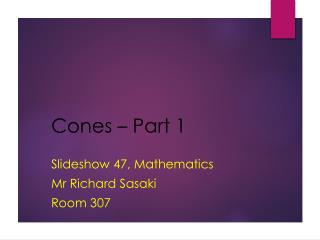DownloadDownload PresentationCones – Part 1

# Cones – Part 1

Download Presentation## Cones – Part 1

- - - - - - - - - - - - - - - - - - - - - - - - - - - E N D - - - - - - - - - - - - - - - - - - - - - - - - - - -
##### Presentation Transcript

1. Cones – Part 1 Slideshow 47, Mathematics Mr Richard Sasaki Room 307

2. Understand the “net” of a cone and its properties • Calculate radii, arc lengths and central angles for sectors and lateral surfaces of cones Objectives

3. Sphere Some Simple 3-D Shapes Cone Cylinder Square-based pyramid Hemisphere

4. Platonic solids / Convex regular polyhedrons Cube Tetrahedron Octahedron Dodecahedron Icosahedron

5. Prisms Cuboid Hexagonal Prism Pentagonal Prism Triangular Prism Octagonal Prism Decagonal Prism Dodecagonal Prism Heptagonal Prism

6. The Net for a Cone We know why a true net for a cone can’t be made…right? It connects at a point with zero size. But what would it look like? Lateral Surface(s) Base(s)

7. The Net for a Cone Let’s look at some cone properties. What will each cone look like? (Thanks Isamu for your help here.)

8. The Net for a Cone Let’s look at the lateral surfaces. Radius / Radii Central angle(s) (Area) Sector(s) (Area) Arc Length

9. Sectors Have a look at the sector below. This would be the cone’s lateral surface. How do we calculate its area and arc length? (Area) For a regular circle… Area = Circumference =

10. Sectors Area = Circumference = If we thought of a circle as a sector, it has a central angle of and an arc length of . A semi-circle would have a central angle of and an arc length of . We divided the area by 2 because the central angle is 180o. How can we write the area in terms of a and r? Area =

11. Sectors Sector Area (S) = (Area) Arc Length () = Example A sector has radius 3cm and central angle 90o. Calculate its area, S and arc length,. S = =

12. Answers tall/thin wide can’t flat face When When

13. The Cone As you know, this sector can be folded to make the lateral face of the cone. (Area) However, on a cone, some information will be represented differently. Slant height -

14. apex base slant height circumference